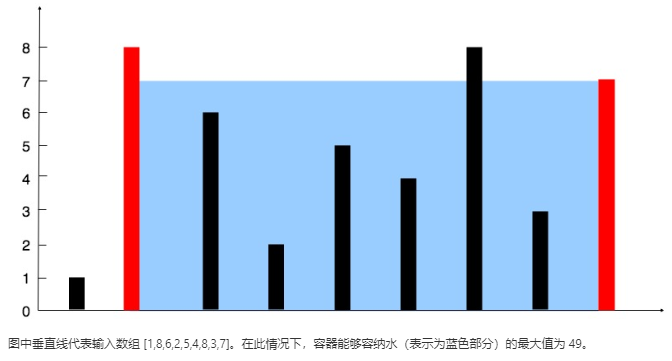# 盛最多水的容器

Leetcode算法题 11 Posted by Haoran Bai on April 17, 2020 · 1 min read

Leetcode 4月每日一题合集

## 1. 题目描述输入：[1,8,6,2,5,4,8,3,7]



## 2. 题解与思路

• l<r 时，就计算当前容器的容量，计算方式为 min(height[l], height[r]) * (r-l)，然后记录当前容量最大值。
• 然后缩小容器，到底该移动哪个指针呢？结论是：应该移动 height 小的指针。

## 3. 代码

class Solution(object):
def maxArea(self, height):
"""
:type height: List[int]
:rtype: int
"""
l, r = 0, len(height)-1  # 左右两个指针
max_water = 0
while l<r:
this_water = min(height[l], height[r]) * (r - l)  # 当前容器容量
max_water = max(max_water, this_water)  # 记录当前容量最大值
if height[l] <= height[r]:  # 移动 height 小的指针
l += 1
else:
r -= 1
return max_water